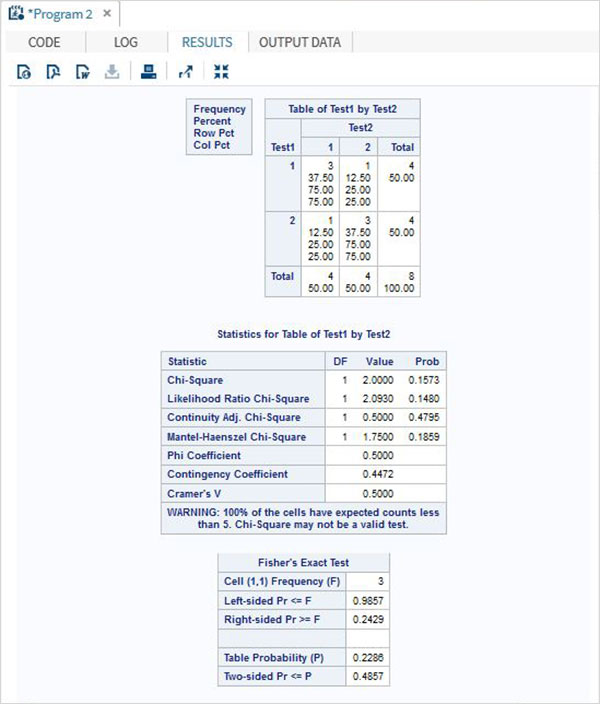# SAS - Fishers Exact Tests

Fisher's exact test is a statistical test used to determine if there are nonrandom associations between two categorical variables.In SAS this is carried out using PROC FREQ. We use the Tables option to use the two variables subjected to Fisher Exact test.

### Syntax

The basic syntax for applying Fisher Exact test in SAS is −

```PROC FREQ DATA = dataset ;
TABLES Variable_1*Variable_2 / fisher;
```

Following is the description of the parameters used −

• dataset is the name of the dataset.

• Variable_1*Variable_2 are the variables form the dataset .

## Applying Fisher Exact Test

To apply Fisher's Exact Test, we choose two categorical variables named Test1 and Test2 and their result.We use PROC FREQ to apply the test shown below.

### Example

```data temp;
input  Test1 Test2 Result @@;
datalines;
1 1 3 1 2 1 2 1 1 2 2 3
;
proc freq;
tables Test1*Test2 / fisher;
run;
```

When the above code is executed, we get the following result −